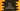# C++ program to find out the sum of factorial series 1! + 2! + 3! + 4!...## C++ program to find out the sum of factorial series 1! + 2! + 3! + 4!…

In this tutorial, we will learn how to find the sum of factorial of a series up to a specific length. Our program will take the value of n from the user and find out the sum. For example, if the value of n is 2, it will find 1! + 2!,it is 5, it will find 1! + 2! +… 5! etc.

With this post, you will learn how to get user inputs and how to find factorial in C++.

## C++ program :

``````#include <iostream>

using namespace std;

int findFact(int n)
{
return n == 1 ? 1 : n * findFact(n - 1);
}

int main()
{
int n, sum = 0;

cout << "Enter the value of n : " << endl;

cin >> n;

for (int i = 1; i <= n; i++)
{
sum += findFact(i);
}

cout << "Sum : " << sum << endl;
}``````

### Explanation:

• findFact method is used to find out the factorial of a number.
• In the main function, we have two int variables n and sum.
• Value of n is taken as user input. Using a for loop, we are finding the factorial of all numbers from 1 to n and adding all values to calculate the final result sum.

### Sample output:

``````Enter the value of n :
4
Sum : 33

Enter the value of n :
10
Sum : 4037913``````

## Method 2: By using the current factorial value:

We can also keep the current factorial value in a variable and multiply that with the next number to get the next value.

``````factorial of 1: 1
factorial of 2: factorial of 1 * 2
factorial of 3: factorial of 2 * 3
factorial of 4: factorial of 3 * 4``````

Below is the C++ program :

``````#include <iostream>

using namespace std;

int main()
{
int n, sum = 0, currentFact = 1;

cout << "Enter the value of n : " << endl;

cin >> n;

for (int i = 1; i <= n; i++)
{
currentFact *= i;
sum += currentFact;
}

cout << "Sum : " << sum << endl;
}``````

Here, we are storing the factorial in currentFact variable. In each iteration of the loop, we are multiplying it with the current value of i to get the factorial for i.